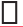# 8. (a)  (i) Provide the number in the box, such that= .                (ii) The simplest form of the number obtained inis _________.     (b)  (i) Provide the number in the box, such that= .                 (ii) The simplest form of the number obtained inis _________.

As we Know, That in the multiplication of fraction, the numerator of both fraction are multiplied to give numerator of new fraction, and denominator of both fractions is multiplied to give the denominator of answer fraction. So,= .

We have to multiply 5 with 2 in the numerator to get numerator equal to 10 and 10 with 3 in the denominator to get 30. So,

The Simplest Form :

.

## Related Chapters

### Preparation Products

##### JEE Main Rank Booster 2021

This course will help student to be better prepared and study in the right direction for JEE Main..

₹ 13999/- ₹ 9999/-
##### Rank Booster NEET 2021

This course will help student to be better prepared and study in the right direction for NEET..

₹ 13999/- ₹ 9999/-
##### Knockout JEE Main April 2021 (Easy Installments)

An exhaustive E-learning program for the complete preparation of JEE Main..

₹ 4999/-
##### Knockout NEET May 2021

An exhaustive E-learning program for the complete preparation of NEET..

₹ 22999/- ₹ 14999/-